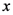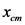# If m2≫m1. then the center of mass is located:Find the x coordinate xcmx_cm of the center of mass of the
system. Express your answer in terms of m1
, m2
, x1
, and x2
.m m x x X_cm= ? 2- If m2?m1 ,
then the center of mass is located:m m If ,
then the center of mass is located:

to the left of m1 at a
distance much greater than x2?x1

to the left of m1 at a
distance much less than x2?x1

to the right of m1 at a
distance much less than x2?x1

to the right of m2 at a
distance much greater than x2?x1

to the right of m2 at a
distance much less than x2?x1

to the left of m2 at a
distance much less than x2?x1
3-
Recall that the blocks can only move along the x axis.
The x components of their velocities at a certain moment
are
and
. Find the component of
the velocity of the center of mass
at that moment. Keep in mind that, in general:
.
Express your answer in terms of m1
, m2
, v1x
, and v2x
. (V_cm)x=
4-
Assume that the x components of the blocks’ momenta at
a certain moment are
and
. Find the x component of the velocity of the center of
mass
at that moment.
,
,
, and
. V_cm=?
5- Assume that the blocks are accelerating, and the x
components of their accelerations at a certain moment are
a1x
and a2x
. Find the x component of the acceleration of the center
of mass (acm)x
at that moment. Keep in mind that, in general,
ax=dvx/dt

.
Express your answer in terms of m1
, m2
, a1x
, and a2x
.